## Motivation

It is important to have an understanding of some of the more traditional approaches to function estimation and classification before delving into the trendier topics of neural networks and decision trees. Many of these methods build on an understanding of each other and thus to truly be a MACHINE LEARNING MASTER, we’ve got to pay our dues. We will therefore start with the slightly less sexy topic of kernel density estimation.

Let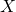be a random variable with a continuous distribution function (CDF)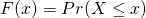and probability density function (PDF)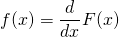Our goal is to estimate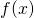from a random sample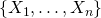. Estimation ofhas a number of applications including construction of the popular Naive Bayes classifier,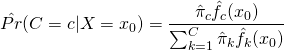## Derivation

The CDF is naturally estimated by the empirical distribution function (EDF)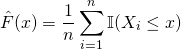where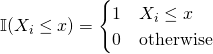I’m not saying naturally to be a jerk! I know the feeling of reading proof-heavy journal articles that end sections with “extension to the d-dimensional case is trivial”, it’s not fun when it’s not trivial to you.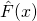essentially estimates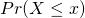, the probability ofbeing less than some threshold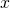, as the proportion of observations in our sample less than or equal to.

It might seem natural to estimate the densityas the derivative ofbutis just a collection of mass points and is not continuous. Instead, lets consider a discrete derivative. For some small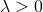,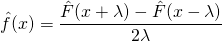This can be re-expressed as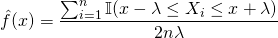since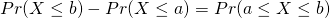(draw a picture if you need to convince yourself)! Simplifying further,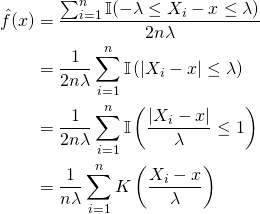where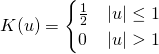is the uniform density function on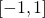. Mathemagic!

From our derivation, we see that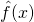essentially determines the number of observations within a small distance,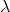, of. The bandwidthdictates the size of the window for which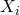are considered. That is, the bandwidth controls the degree of smoothing. The greater the number of observations within this window, the greateris.

Our derived estimateis a special case of what is referred to as a kernel estimator. The general case is

(1)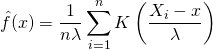where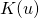is a kernel function.

## Kernel Functions

A kernel function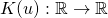is any function which satisfies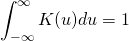The kernel function acts as our weighting function, assigning less mass to observations farther from. This helps to ensure that our fitted curve is smooth.

Non-negative kernels satisfy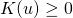for all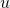and are therefore probability density functions. Symmetric kernels satisfy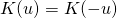for all. The Gaussian, or Normal, distribution is a popular symmetric, non-negative kernel.

The moments of a kernel are defined as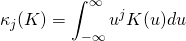The order of a kernel,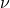, is defined as the order of the first non-zero moment. For example, if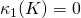and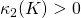, then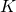is a second-order kernel and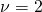. Symmetric non-negative kernels are second-order and hence second-order kernels are the most common in practice.

Other popular kernels include the Epanechnikov, uniform, bi-weight, and tri-weight kernels. The Epanechnikov kernel is considered to be the optimal kernel as it minimizes error. Choice of the bandwidth, however, is often more influential on estimation quality than choice of kernel.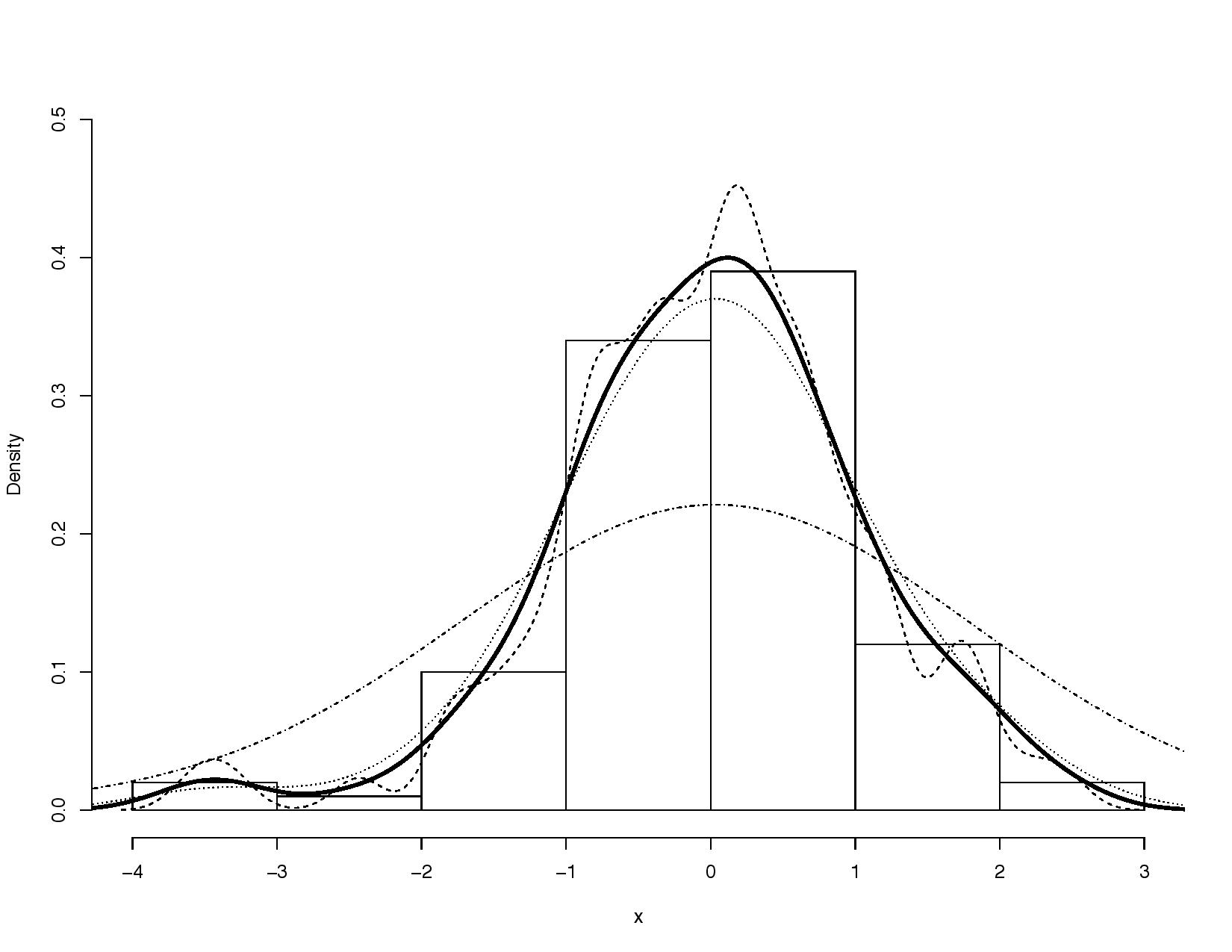Kernel density estimates for various bandwidths. The thick black line represents the optimal bandwidth, . The jagged dotted line is the estimate of when the bandwidth is halved, . The flatter, bell-shaped curve represents which clearly oversmooths the data.

## Bandwidth Considerations

As noted above, the bandwidthdetermines the size of the envelope aroundand thus the number of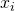used for estimation. In the case of a Gaussian kernel,would translate to the standard deviation. For k-nearest neighbours,would translate to the size of the neighbourhood expressed as the span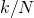(k points within the N observation training set).

The infamous bias-variance trade-off must be considered when selecting. If we choose a small value of, we consider a smaller number of. This results in higher variance due to smaller sample size but less bias as eachwill be closer to. As we increase, our window size increases and we consider a larger number of. This reduces our variance but our bias will now be higher as we are usingthat are further fromand thus information that might not be particularly relevant.

In other words, ifis too large we will smooth out important information but if it is too small, our estimate will be too rough and contain unnecessary noise. Choosingis no easy task and several methods for bandwidth selection have been proposed including cross-validation methods, rules of thumb, and visual inspection.

Personally, I prefer to use cross-validation as a starting point since I try to minimize the effect of my own biases on estimation. However, these methods aren’t perfect and if feasible, I will follow this up with visual inspection to ensure that the CV bandwidth makes sense in the context of my data and problem. I will generally select a slightly rougher fit over a smoother fit as it is easier for the human eye to imagine a smoother fit than a rougher fit!

## Properties of the Kernel Density Estimator

The kernel density estimator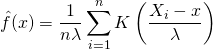has several convenient numerical properties:

1. If the kernel functionis non-negative, the density estimatoris also non-negative.
2.integrates to one, making it a valid density function whenis non-negative.

To prove this, let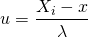Then, via a change-of-variables,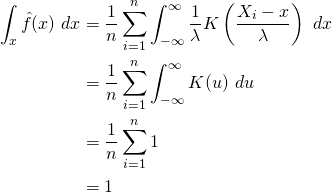3. The mean of the estimated density is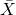.

Using the following transformation,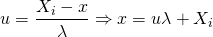and thus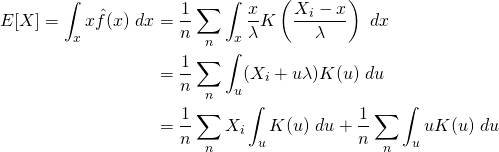Recall that the kernel functionmust integrate to one and that for second-order kernels,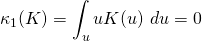Therefore,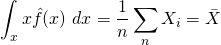4. The variance of the estimated density is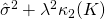. That is, the sample variance is inflated by a factor of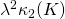when the density is estimated.

The variance ofis given by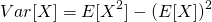The second moment of the estimated density is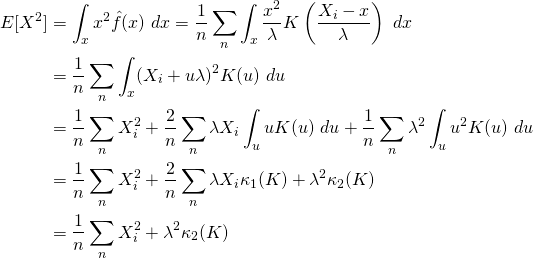Thus,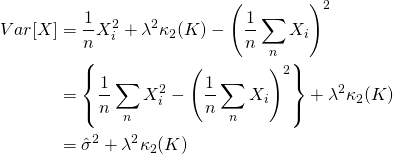## Summary

• The empirical distribution function (EDF) assigns a mass of 1/N to each, resulting in a discrete or “jumpy” estimate.
• Kernel density estimators (KDE) estimateby constructing a neighbourhood around the point of interest. Observations within this neighbourhood are then assigned a mass based on their distance fromvia a kernel function, resulting in a smooth estimate.
• Popular kernel choices are the Gaussian and Epanechnikov kernels. These kernels are second-order kernels, suggesting they are both proper, symmetric density functions.
• The size of the neighbourhood is dictated by the bandwidth. Special care must be taken when selectingin order to ensure that the bias-variance trade-off is balanced for your problem. Different methods such as CV are available to assist you with optimal bandwidth selection.
• Choice of bandwidth has a larger impact on estimation quality than your choice of kernel.

I will be extending the kernel density estimator to kernel regression in my future blog posts and conducting a case study in R that uses these methods, stay tuned!